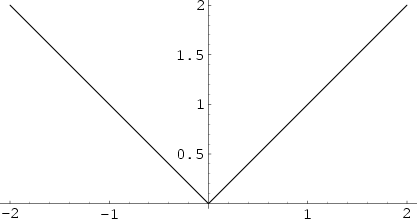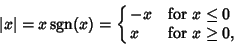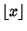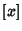## Absolute ValueThe absolute value of a Real Numberis denotedand given bywhere Sgn is the sign function.

The same notation is used to denote the Modulus of a Complex Number,, a p-adic Number absolute value, or a general Valuation. The Norm of a Vectoris also denoted, althoughis more commonly used.

Other Notations similar to the absolute value are the Floor Function, Nint function, and Ceiling Function.

See also Absolute Square, Ceiling Function, Floor Function, Modulus (Complex Number), Nint, Sgn, Triangle Function, Valuation